Next: Gravity Waves in Shallow Up: Waves in Incompressible Fluids Previous: Gravity Waves

# Gravity Waves in Deep Water

Consider the so-called deep water limit,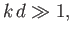(11.22)

in which the depth,, of the water greatly exceeds the wavelength,, of the wave. In this limit, the gravity wave dispersion relation (11.21) reduces to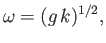(11.23)

because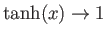as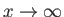. It follows that the phase velocity of gravity waves in deep water is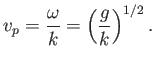(11.24)

Note that this velocity is proportional to the square root of the wavelength. Hence, deep-water gravity waves with long wavelengths propagate faster than those with short wavelengths. The phase velocity,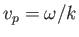, is defined as the propagation velocity of a plane wave with the definite wave number,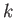[and a frequency given by the dispersion relation (11.23)] (Fitzpatrick 2013). Such a wave has an infinite spatial extent. A more realistic wave of finite spatial extent, with an approximate wave number, can be formed as a linear superposition of plane waves having a range of different wave numbers centered on. Such a construct is known as a wave pulse (Fitzpatrick 2013). As is well known, wave pulses propagate at the group velocity (Fitzpatrick 2013),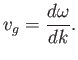(11.25)

For the case of gravity waves in deep water, the dispersion relation (11.23) yields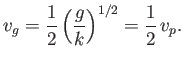(11.26)

In other words, the group velocity of such waves is half their phase velocity.

Let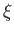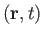be the displacement of a particle of water, found at position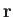and time, due to the passage of a deep water gravity wave. It follows that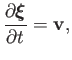(11.27)

whereis the perturbed velocity. For a plane wave of wave number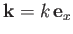, in the limit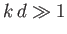, Equation (11.19) yields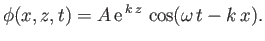(11.28)

Hence, [cf., Equations (11.45)-(11.48)]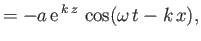(11.29)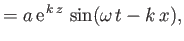(11.30)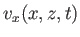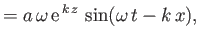(11.31)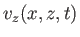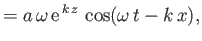(11.32)

and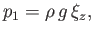(11.33)

where use has been made of Equations (11.7), (11.9), and (11.27). Here,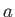is the amplitude of the vertical oscillation at the water's surface. According to Equations (11.29)-(11.32), the passage of the wave causes a water particle located a depthbelow the surface to execute a circular orbit of radius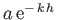about its equilibrium position. The radius of the orbit decreases exponentially with increasing depth. Furthermore, whenever the particle's vertical displacement attains a maximum value the particle is moving horizontally in the same direction as the wave, and vice versa. (See Figure 11.1.)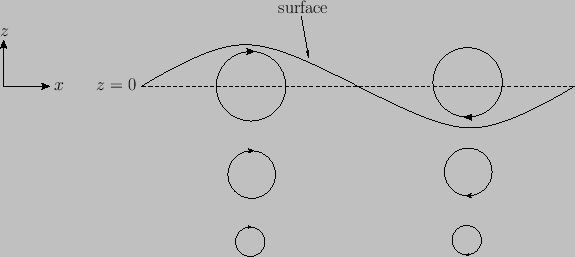Finally, if we define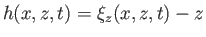as the equilibrium depth of the water particle found at a given point and time then Equations (11.3) and (11.33) yield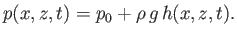(11.34)

In other words, the pressure at this point and time is the same as the unperturbed pressure calculated at the equilibrium depth of the water particle.Next: Gravity Waves in Shallow Up: Waves in Incompressible Fluids Previous: Gravity Waves
Richard Fitzpatrick 2016-03-31